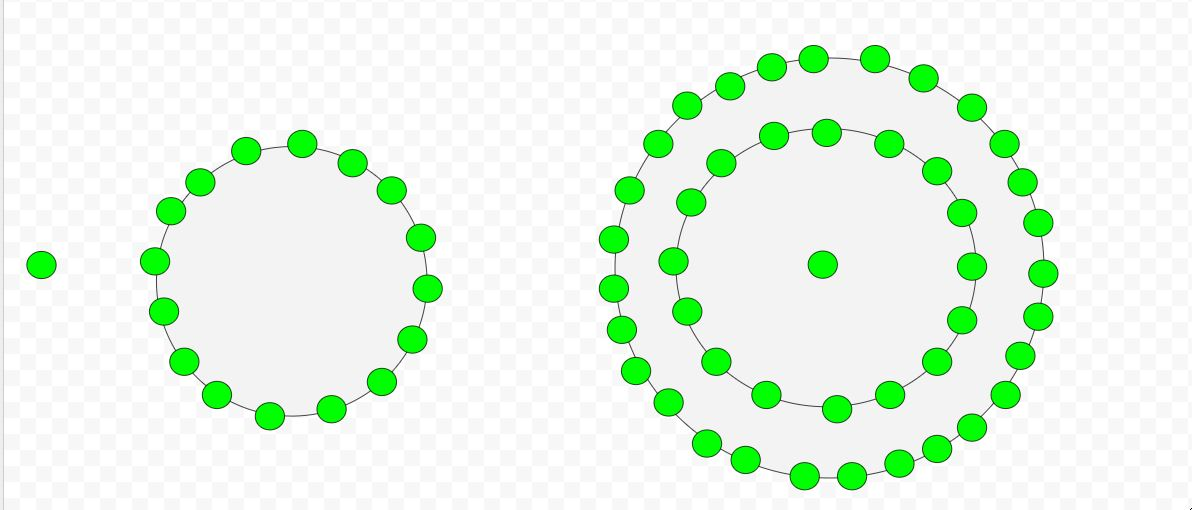• Last Updated : 06 Sep, 2021

Given a number n, the task is to find the nth hexadecagonal number.
A Hexadecagonal number is class of figurate number and a perfect squares. It has sixteen sided polygon called hexadecagon or hexakaidecagon. The n-th hexadecagonal number count’s the sixteen number of dots and all others dots are surrounding to its successive layer.
Examples :

Input : 2
Output :16
Input :7
Output :301

Attention reader! Don’t stop learning now. Get hold of all the important DSA concepts with the DSA Self Paced Course at a student-friendly price and become industry ready.  To complete your preparation from learning a language to DS Algo and many more,  please refer Complete Interview Preparation Course.

In case you wish to attend live classes with experts, please refer DSA Live Classes for Working Professionals and Competitive Programming Live for Students.## C++

 // C++ program to find Nth// hexadecagon number#include using namespace std;  // Function to calculate hexadecagonal numberint hexadecagonalNum(long int n){    return ((14 * n * n) - 12 * n) / 2;}  // Drivers Codeint main(){    long int n = 5;    cout << n << "th Hexadecagonal number : ";    cout << hexadecagonalNum(n);    cout << endl;    n = 9;    cout << n << "th Hexadecagonal number : ";    cout << hexadecagonalNum(n);      return 0;}

## Java

 // Java program to find Nth hexadecagon// numberimport java.io.*;  class GFG {      // Function to calculate hexadecagonal    // number    static long hexadecagonalNum(long n)    {        return ((14 * n * n) - 12 * n) / 2;    }          // Drivers Code    public static void main (String[] args)    {        long n = 5;        System.out.println( n + "th "          + "Hexadecagonal number : "              + hexadecagonalNum(n));                        n = 9;        System.out.println( n + "th "          + "Hexadecagonal number : "              + hexadecagonalNum(n));    }}  // This code contributed by anuj_67.

## Python3

 # Python program to find Nth# hexadecagon number  # Function to calculate# hexadecagonal numberdef hexadecagonalNum(n):      # Formula to calculate nth    # Centered hexadecagonal number    return ((14 * n * n) - 12 * n) // 2  # Driver Coden = 5print("%sth Hexadecagonal number : " %n,                    hexadecagonalNum(n))n = 9print("%sth Hexadecagonal number : " %n,                    hexadecagonalNum(n))                      # This code is contributed by ajit

## C#

 // C# program to find Nth hexadecagon// numberusing System;class GFG {      // Function to calculate hexadecagonal    // number    static long hexadecagonalNum(long n)    {        return ((14 * n * n) - 12 * n) / 2;    }          // Drivers Code    public static void Main ()    {        long n = 5;        Console.WriteLine( n + "th "        + "Hexadecagonal number : "            + hexadecagonalNum(n));                      n = 9;        Console.WriteLine( n + "th "        + "Hexadecagonal number : "            + hexadecagonalNum(n));    }}  // This code contributed by anuj_67.

## PHP

 

## Javascript

 

Output :

5th Hexadecagonal number : 145
9th Hexadecagonal number : 513

Time Complexity: O(1)
Auxiliary Space: O(1)
Reference: https://en.wikipedia.org/wiki/Polygonal_number

My Personal Notes arrow_drop_up